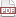### Tip 58: Destroy your inputs

26 January 12. [link]PDF version

level: function writer
purpose: use the declarations you already have

Part of a series of tips on POSIX and C. Start from the tip intro page, or get 21st Century C, the book based on this series.

This is not a new tip. In fact, it's on page 24 of the 1ed. of K&R:

Call by value is an asset, however, not a liability. It usually leads to more compact programs with fewer extraneous variables, because arguments can be treated as conveniently initialized local variables in the called routine.

I really hope this is an ex post justification for the system, not their real motivation, because the technique of screwing around with the input variables knowing they can't affect the main program has somewhat limited utility.

Integers can often be used as countdown variables. To rewrite K&R's example:

double power(double x, int n){ //assume n>=0
double out =1;
for (; n>0; n--) out *= x;
return out;
}

int main(){
printf("2^5: %g\n", power(2, 5));
printf("2.5^5: %g\n", power(2.5, 5));
printf("3^5: %g\n", power(3, 5));
}


If you have a NULL-terminated list, you already have what you need to step through it. We guaranteed such a list in Tip 26, so let's rewrite the code there without bothering with an index in the sum_base function:

#include <math.h> //NAN
#include <stdio.h>

#define sum(...) sum_base((double[]){__VA_ARGS__, NAN})

double sum_base(double  in[]){
double out=0;
for ( ; !isnan(*in); in++) out += *in;
return out;
}

int main(){
double two_and_two = sum(2, 2);
printf("2+2 = %g\n", two_and_two);
printf("(2+2)*3 = %g\n", sum(two_and_two, two_and_two, two_and_two));
printf("sum(asst) = %g\n", sum(3.1415, two_and_two, 3, 8, 98.4));
}


The code isn't actually shorter, but the for loop uses the input pointer to step through the array, rather than using an index to step through. If we had a linked list, then it may be impossible to use the index-based form, so you're forced to use a form like this one.

This may come up frequently in main, because argv is just the sort of list you would want to step through--and the standard says that its last element (argv[argc]) is NULL (C99 & C11 §5.1.2.2.1.2, which also says that you are allowed to modify the values of argv in place--they are not string constants). So, in fact, argc may be entirely redundant.

OK, you can count down with integers, and you can use pointers to arrays or lists to step through the structure and check up on each element individually. Are there any other ways to take advantage of inputs as conveniently initialized variables?